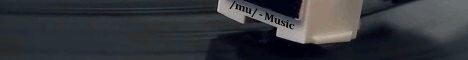[a / b / c / d / e / f / g / gif / h / hr / k / m / o / p / r / s / t / u / v / vg / vm / vmg / vr / vrpg / vst / w / wg] [i / ic] [r9k / s4s / vip / qa] [cm / hm / lgbt / y] [3 / aco / adv / an / bant / biz / cgl / ck / co / diy / fa / fit / gd / hc / his / int / jp / lit / mlp / mu / n / news / out / po / pol / pw / qst / sci / soc / sp / tg / toy / trv / tv / vp / vt / wsg / wsr / x / xs] [Settings] [Search] [Mobile] [Home]
Board
[s4s] - Sh*t 4chan Says>>
>>10586134
uhhhhhhh 24
>>
>>10586134
bury elitist

>>
>>10586134
2
>>
i could answer it but i'm too lazy to type it into wolfram alpha
>>
thank me later virgin

Your fortune: Better not tell you now
>>
>>10586146
you can solve this with python btw
>>
>>10586150
my pocket calculator can solve it too

i could do it in assembly if i wanted

feeling kinda chad tbh because most zoomers these days probably dont even know what that calculus swoosh is :^)
>>
you can also do it analytically by noticing one of the terms is odd and so evaluates to 0, and what's left is just an expression for half the area of a semicircle of radius 2
>>
>>10586184
totally thats what i did and now i have free wifi
>>
shouldnt the dx be not under the root symbol?
>>
>>10586270
I noticed that too. Whoever made this sign is an retard.
>>
answer is pi. you can read it off without needing any calculations if you are comfortable enough with calculus. x^3 cos(x/2) are both odd functions so their product is an odd function too so the term involving it is equal to 0, which just leaves 1/2sqrt(...), this is just a half-circle of radius 2 so the area must be (1/2)((pi * 2^2)/2) which is equal to pi
>>
>>10586270
>>10586743
trolled hard
t: maker of the sign
>>
>>10586756
every word of this requires so much background knowledge that it'd probably take me months or years to actually even parse half of what you're saying

>what is an odd (or even) function
>why is x^3 an odd function
>why is cos(x/2) an odd function
>why are they even functions? aren't they results of functions? or are the cube and cosine the functions?
>what is a cosine or a sine
>how do you know how to calculate a cosine without putting it in a calculator/how do you know a cosine is odd or whatever
>what does it mean to multiply functions
>why is the product of odd functions an odd function
>what is a "term" (here, and in general)
>why are odd function terms (?) 0
>why is half of a square root a half-circle of radius 2
>what is the relationship between the square root and the circle, or anything else and the circle. what causes one to believe a circle is involved here. the cosine? how does a cosine relate to a circle. or I thought that doesn't matter anymore since you said "which just leaves 1/2sqrt(...)"
>why would one think any area of anything is involved
>why is the area of a half-circle of radius 2 half of pi times 2 squared divided by 2, how does one remember any of that with so many different parts
>what's the squiggly symbol with the 2. does that mean area? what do the 2s mean?
>what's the dx have to do with it (that means something to do with derivatives right? what even is a derivative)
>why did your answer not directly mention the 4 or the subtraction
>where does the x squared come in

and like 50 other things. (I'm a well-paid programmer but I don't know how to do any math and don't know what a function is besides the programming sense of one)
>>
>>10586768
how do you not know what a cosine or sine is

i thought everyone instantly intuitively understands it upon being born
>>
it's their entire reason for existing
>>
>>10586809
where are the PoCs?
>>
>>10586134
it's scary how fast you forget the details of solving integrals. still the sign is dumb because they put dx under the root and tell that the password is the first digits? meaning the first 2 digits, the first 3, 4?
>>
>>10586756
anonymous! cos(x) is an even function and (odd x odd)(x) is even! but (odd*even) is odd so you are right anyway
>>10586156
how much does it take in assembly? just use python trust me every math enthusiast is a CHAD
>>10586768
this is why every software you buy is bugged lole

>>
>>10587544
>how much does it take in assembly?

it'll be ez. the integral can be solved by a square integral.
cos and sqrt are machine level instructions.

now on the other hand if you were to do it in brainfuck - that would be messy. i challenge someone to be an absolute madman and do it. (and no, simply printing the answer because you already know it, doesnt count.)
>>

▲▲

this is all you need

Delete Post: [File Only] Style: